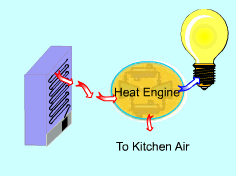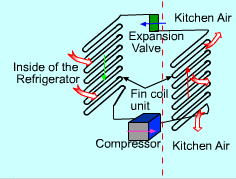Ch 5. Second Law of Thermodynamics Multimedia Engineering Thermodynamics Heat Engine The SecondLaw CarnotCycle Carnot HeatEngine CarnotRefrigerator
 Chapter 1. Basics 2. Pure Substances 3. First Law 4. Energy Analysis 5. Second Law 6. Entropy 7. Exergy Analysis 8. Gas Power Cyc 9. Brayton Cycle 10. Rankine Cycle Appendix Basic Math Units Thermo Tables Search eBooks Dynamics Fluids Math Mechanics Statics Thermodynamics Author(s): Meirong Huang Kurt Gramoll ©Kurt GramollTHERMODYNAMICS - CASE STUDY IntroductionProblem Description A refrigerator in a kitchen dissipates heat to the kitchen air through the fin coil unit located on the back of the refrigerator. If a heat engine is operating between the fin coil unit and the surrounding air, can it generate power? What is known: The coefficient of performance (COP) of the refrigerator is 3.0. The power input to the refrigerator is 1.0 kW. The average surface temperature of the fin coil unit is 30 oC. The kitchen air temperature is 20oC. QuestionHow a Refrigerator Works Determine the maximum power generated by the heat engine operating between the the fin coil unit and the kitchen air. Approach Determine the heat dissipated from the the fin coil unit, which is the heat input to the heat engine. Assume the heat engine is a Carnot heat engine. Its thermal efficiency is determined by ηth,rev. = 1 - TL/TH